Next: Calabi-Yau compactifications Up: SUPERSTRING PHENOMENOLOGY BEFORE 1995 Previous: Toroidal Compactifications

## Orbifold Compactifications

We have then succeeded in constructing 4D superstring models from toroidal compactifications and understand the full class of these models given by the moduli space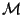. Unfortunately, all of these models have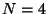supersymmetry and therefore they are not interesting for phenomenology, because they are not chiral. To obtain a chiral model we should construct models with at most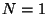supersymmetry. If we still want to use the benefits of free 2D theories, we should construct models from flat space and modify only the boundary conditions. We have already considered identifications by shift symmetries of a lattice defining the tori. We still have the option to also use rotations and consider twisted' boundary conditions . As an example let us start with the torus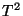discussed before. If we make the identification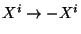we are constructing the orbifold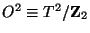, shown in figure 2, where the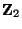twist is rotation by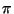. This space is not a manifold because it is singular at the points left fixed by the rotation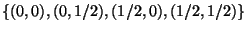. Notice that, for instance, the point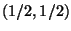is fixed because it is transformed to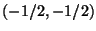which is identical to the original point after a lattice shift. In general, the discrete group of rotations defining the orbifold is called the point group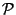, whereas the nonabelian group including the rotations and also the translations of the lattice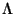, is the space group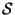. So usually a torus is defined as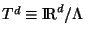and an orbifold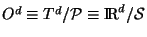.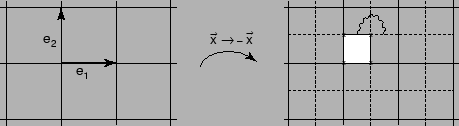We can easily construct 4D strings from orbifold compactifications in which the 10D spacetime of the heterotic string is the product of 4D flat spacetime and a six-dimensional orbifold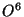. The heterotic string is particularly interesting because we can extend the action of the point group to the 16D lattice of the gauge group by embedding the action of the orbifold twist in the gauge degrees of freedom defined by the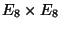lattice, say. This can easily be done in two ways:

(i) Perform a homomorphism of the point group action in the gauge lattice by shifting the lattice vectors by a vector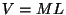where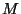is the order of the point group and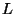is any lattice vector in 16D.

(ii) Perform the homomorphism by twisting also the gauge lattice by an orderrotation belonging to the Weyl group of the corresponding gauge group.

These embeddings on the gauge degrees of freedom allow us to break the gauge group, reduce the number of supersymmetries and generatechiral models in 4D as desired. The reason for this is the following: using the embedding by a shift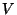, we start with the spectrum of the toroidal compactification and have to project out all the states that are not invariant by the orbifold twist. For the gauge group, only the elements satisfying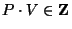remain, where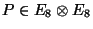, breaking the gauge group to a subgroup of the same rank. The four gravitinos of thetoroidal compactification also transform and depending on the orbifold twist they are reduced to only one or two invariant states, indicating that there is only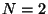orsupersymmetry. Actually there are only four twists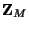leading to(for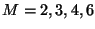) and some twentyor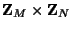twists leading tosupersymmetry , which are the phenomenologically interesting ones. For each of these twists we can have several (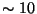) different embeddings on the gauge degrees of freedom. One of these embeddings is called the standard embedding because it acts identically in the gauge degrees of freedom as in the 6D space, this embedding also describes compactifications of the type II strings and is distinguished because in the 2D worldsheet, the corresponding model has two supersymmetries on the left-movers and two supersymmetries on the right-movers, the corresponding models are called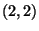models. All other embeddings do not have supersymmetry in the left moving side and are called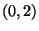models.

On top of all these embeddings we can also add Wilson lines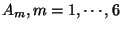, by embedding the shifts of the lattice defining the 6D compactified torus, on the gauge degrees of freedom in terms of further shifts of the 16D gauge lattice, which will further break the gauge group. This increases the number of possible consistent models by a large amount, which we can only estimate between millions and billions because some may turn out to be actually equivalent. The Wilson lines can also be interpreted as the full embedding of the space groupin the gauge degrees of freedom. In this case, using the two alternative embeddings mentioned above will give a completely different result because in the first case, bothand the Wilson lines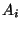will act as shifts and so the embedding is abelian, whereas in the second option we will have both shifts and twists so the embedding is non-abelian. This possibility allows for two important properties: the Wilson lines are continuous rather than quantized and the rank of the gauge group can be reduced. In the absence of Wilson lines both embeddings are equivalent. Both classes of embeddings can be obtained by starting with the Narain lattice of toroidal compactifications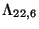and twist it in a consistent manner. This already takes into account the discrete and continuous Wilson lines (which were already present, parametrizing) and also allows for the possibility of performing left-right asymmetric twists, the so-called asymmetric orbifolds . This extra degree of freedom increases the number of possible models.

We can see now how a vast amount of heterotic string orbifold models can be generated. There are many (billions?) classes of models; different classes differentiated by the choice of original 6D toroidal lattice, the orbifold point group, the embeddingsand the discrete Wilson lines. But each of these discrete choices allows for a variation of different continuous parameters such as the moduli fields (like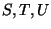), the continuous Wilson lines (that correspond to charged untwisted sector moduli fields) and there are also charged twisted-sector moduli fields. All the continuous parameters can be seen as flat potentials for fields in the effective field theoretical effective action.

Only a few of these classes of models includes quasi-realistic models. As an example , the model based on the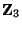orbifold with embeddingand nonvanishing Wilson lines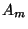given by: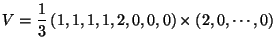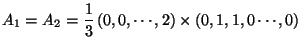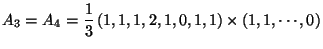(12)

breaksto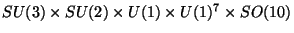with three families of quarks and leptons. The extra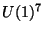symmetry can be broken by the standard Higgs mechanism which, in string theory requires the existence of flat directions among some charged matter fields; these can be analyzed in the models at hand because there are general selection rules' forbidding couplings not invariant under the action of the point and space groups. The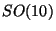remains as a hidden sector (in the sense that it only has gravitational strength couplings with the observable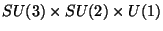sector). This is an example of a quasi realistic model. The structure of Yukawa couplings can be analyzed leading to very realistic properties and problems such as very fast proton decay can be avoided. However, there are extra doublets in the model that give rise to unrealistic values of Weinberg's angle. This may in principle be solved by contemplating the existence of intermediate scales, but at this point the model stops being stringy. It also has the drawback that without knowing details about supersymmetry breaking many of the low energy parameters can not be determined. There are variations of this model that allow for an extra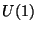symmetry at low energies, implying a relatively light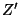particle. There are several models in the literature with similar properties as this one, showing that it is possible to get models very close to the standard model of particle physics. But there is not a single model that could be considered realistic. In particular there is no model yet with just the spectrum of the supersymmetric standard model.Next: Calabi-Yau compactifications Up: SUPERSTRING PHENOMENOLOGY BEFORE 1995 Previous: Toroidal Compactifications
root 2001-01-22